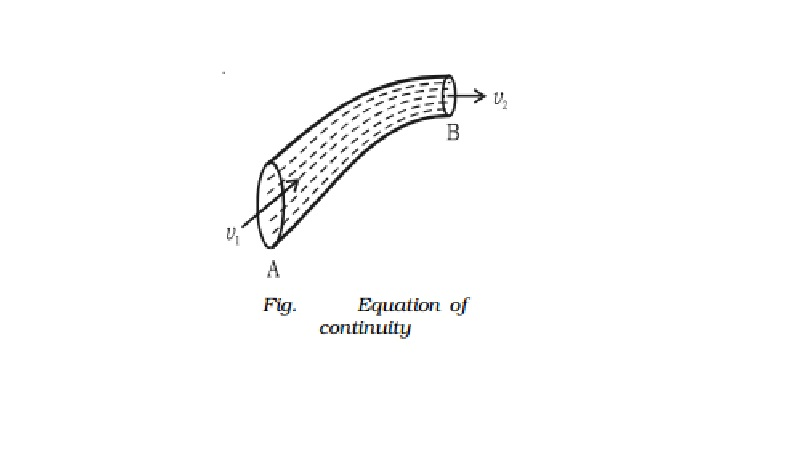Home | | Physics | | Physics | Fluid Equation of continuity

# Fluid Equation of continuityConsider a non-viscous liquid in streamline flow through a tube AB of varying cross section as shown in Fig. Let a1 and a2 be the area of cross section, v1 and v2 be the velocity of flow of the liquid at A and B respectively.

Equation of continuity

Consider a non-viscous liquid in streamline flow through a tube AB of varying cross section as shown in Fig.  Let a1 and a2 be the area of cross section, v1 and v2 be the velocity of flow of the liquid at A and B respectively.

Volume of liquid entering per second at A = a1v1.

If ρ is the density of the liquid, then mass of liquid entering per second at A = a1v1ρ.

Similarly, mass of liquid leaving per second at B = a2v2ρ

If there is no loss of liquid in the tube and the flow is steady, then mass of liquid entering per second at A = mass of liquid leaving per second at B(i.e) a1v1ρ = a2v2ρ or a1v1 = a2v2 i.e. av = constant

This is called as the equation of continuity. From this equation

v α a1 .

i.e. the larger the area of cross section the smaller will be the velocity of flow of liquid and vice-versa.

Total energy of a liquid

A liquid in motion possesses pressure energy, kinetic energy and potential energy.

(i) Pressure energy

It is the energy possessed by a liquid by virtue of its pressure.

(ii) Kinetic energy

It is the energy possessed by a liquid by virtue of its motion.

If m is the mass of the liquid moving with a velocity v, the kinetic energy of the liquid = � mv2

(iii) Potential energy

It is the energy possessed by a liquid by virtue of its height above the ground level.

If m is the mass of the liquid at a height h from the ground level, the potential energy of the liquid = mgh

Study Material, Lecturing Notes, Assignment, Reference, Wiki description explanation, brief detail

Related Topics